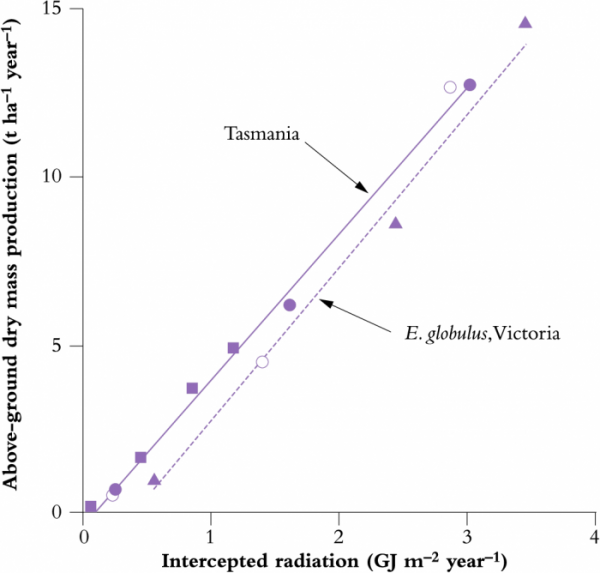# 12.3.2 - Canopy productivity

Photosynthesis is driven by the fraction of radiation intercepted by the canopy and gross photosynthetic production ($A_g$), a measure of the total amount of CO2 ﬁxed in photosynthesis, can be expressed as:

$A_g = A_0 [1 - exp(-kS_{a}W_{l})] \tag{12.4}$

$A_0$ is the gross photosynthetic production at full light interception and $S_{a}W_{l}$ expresses LAI as the product of speciﬁc leaf area ($S_{a}$, the ratio of leaf area:leaf dry mass) and dry mass of leaf organic matter ($W_{l}$, often approximated as leaf dry mass).

### Efficiency of light conversion to biomass ($\varepsilon$)

As implied by Equation 12.4, there is a proportional relationship between production of dry mass and interception of radiation (see Fig. 12.22), while LAI is a major determinant of photosynthetic production. The slope of this relationship is a measure of the conversion efﬁciency ($\varepsilon$) of light (photon irradiance if based on PAR) or irradiance ($I$ if based on shortwave radiation) to dry mass. $\varepsilon$ has units of g MJ–1 and values based on photon irradiance are approximately twice those based on $I$. $\epsilon$ can be considered to be the canopy-scale equivalent of $\varphi$, the quantum yield of individual leaves. Values of $\varepsilon$ based on absorbed radiation are net of any light or radiation that is reflected upward from the direction in which the incident value is measured.

This proportional relationship was ﬁrst clearly deﬁned in the above terms for the seasonal growth of temperate agricultural and horticultural crops in Britain (Monteith 1977, Fig. 12.22). It has since been shown to hold for a range of vegetation types and environments. Proportionality occurs because photosynthesis by most leaves in a canopy tends to be light limited. Consequently any increase in light intercepted or absorbed results in an increase in dry mass production. As crops grow from establishment or plant communities develop from a state of initial colonisation to maturity, LAI increases, and a greater leaf surface results in greater levels of light interception and rates of growth.

## 12.3-Ch-Fig-12.26.pngFigure 12.26 Above-ground dry mass production and intercepted radiation for eucalypts in plantations in Tasmania and Victoria show a linear relationship. Closed symbols refer to different species or provenances of the same species at Tasmanian sites. Open symbols refer to Eucalyptus globulus growing in Victoria. (Original data C.L. Beadle and G. Inions)

As predicted by theory, linear relationships between above-ground dry mass production and intercepted radiation have been observed for eucalypts in plantation forests in southeast Australia: $\varepsilon$ was around 0.45 g MJ–1 (based on $I$, Figure 12.26). Conversion efﬁciency was independent of species and provenance within species, and for one species, Eucalyptus globulus, was independent of site. Differences in growth rate between species, in this case during the early phase of forest growth, can be entirely a function of more rapid development of LAI in one species compared to another.

Comparative analyses of $\varepsilon$ for different vegetation types are commonly based on above-ground dry mass ($W_{a}$) because of lack of information on below-ground biomass ($W_{b}$). $\varepsilon$ based on $W_{a}$ will be less than that based on $W_{a} + W_{b}$ by the ratio $W_{a} : (W_{a} + W_{b})$. Partitioning of dry mass to roots may be substantially higher on a resource-poor compared to a resource-rich site. Consequently, a comparison of $\varepsilon$ between sites based on $W_{a}$ rather than $W_{a} + W_{b}$ would lead to a relative underestimate of the efﬁciency of conversion of light to dry mass on poorer sites. Similarly, stress in response to soil water deﬁcit, high vapour pressure deﬁcit (leading to stomatal closure) or extremes of temperature will reduce $\varepsilon$ and may also change the partitioning of dry mass. Reductions in $\varepsilon$ occur in response to stomatal closure or to stresses of sufﬁcient severity to reduce the quantum yield of photosynthesis. In effect, plants reduce photosynthesis by redirecting absorbed energy away from photochemistry and into photoprotective pathways which disperse absorbed energy as heat. $\varepsilon$ thus embodies the photosynthetic history of a crop over a given interval and integrates the effects of all environmental variables on photosynthetic utilisation of absorbed radiation.

In summary, forest canopies consist of multiple layers. A logarithmic gradient of sunlight availability exists from upper to lower layers, and leaf properties adjust accordingly.